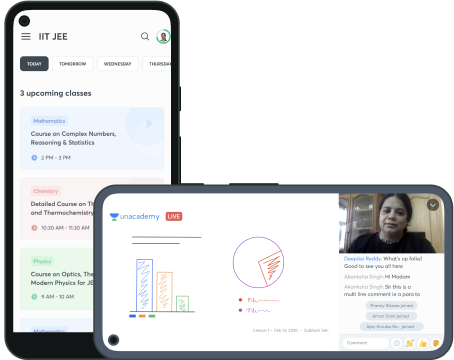# FREE Course on Probability and Expected Values

In this course, you will learn what is probability and expected values. Why are they relevant? Then learn various techniques to solve expected value problems. You will also be provided with solved examples and practice problems.

#### Probability and Expected Values - I

In this session, Triveni will define the basic terminologies in probability and expected values. And, understand them well with examples. This is session is pre-requisite for the next 3 sessions.

Apr 19, 2021 • 2h 0m

Triveni Mahatha

#### Probability and Expected Values - II

In this session, Triveni will use the basic definitions and techniques to solve example problems on expected values. How to use recurrence relations?

Apr 20, 2021 • 2h 0m

Triveni Mahatha

#### Probability and Expected Values - III

In this session, Triveni will introduce the concept of linearity of expectations. And, use this technique to solve problems. This is the most used and very powerful technique.

Apr 21, 2021 • 2h 0m

Triveni Mahatha

#### Probability and Expected Values - IV

In this session, Triveni will solve more interesting and advanced problems using known techniques like DP, Linearity of expectations, recurrence relations, basic definitions etc.

Apr 22, 2021 • 2h 0m

Triveni Mahatha